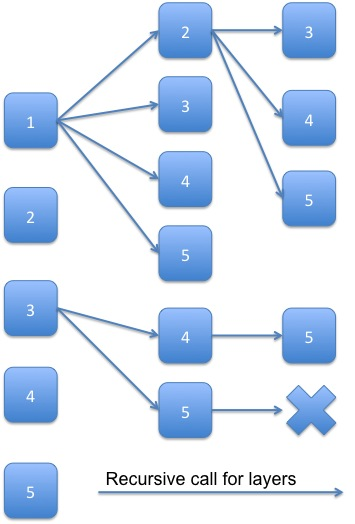# [leet code] Combinations

Given two integers n and k, return all possible combinations of k numbers out of 1 ... n.

For example,
If n = 4 and k = 2, a solution is:

[
[2,4],
[3,4],
[2,3],
[1,2],
[1,3],
[1,4],
]

=================

Note that in each combination, former number is smaller than the following number.  In another word, [1, 2] and [2, 1] is considered as the same combination, and only [1, 2] is required as the result in this problem.

As usual, if we don't have any idea about the solution, we can try to manually process 1-2 examples.  Here is an example I tried, n=5, k=3:From this example, we can see that in the first position of the resulting combinations we can chose number 1-5.  Assume that we chose 1 for the 1 position of the combination, then we can choose 2-5 for the second position.  Till we chosen numbers for all position, we can have one possible combination.

However, when we chose 3 for the first position and 5 for the second position, we don't have any other number to choose for the 3rd position. (former number must be smaller than the following number).

Now we have the rules of this problem, and we can try to implement these rules.  For choosing number in a specific layer, we can use a iteration, e.g. in the first layer, we try 1-5.  While for step in another layer, we can use recursive call.  Parameters we need for a recursive call are the current combination, the combination result set, the n, the k, and the start point of iteration (e.g. if we chose 1 for the first position, start point of 2nd position is 2; while if we chose 2 for the first position, start point of second position is 3).

public class Solution {
public ArrayList<ArrayList<Integer>> combine(int n, int k) {
ArrayList<ArrayList<Integer>> combSet = new ArrayList<ArrayList<Integer>>();
ArrayList<Integer> comb = new ArrayList<Integer>();

if(n<k) return combSet;
helper(n, k, combSet, comb, 1);
return combSet;
}

public void helper(int n, int k, ArrayList<ArrayList<Integer>> combSet, ArrayList<Integer> comb, int start){
// one possible combinition constructured
if(comb.size() == k){
return;
}

else{
for(int i=start; i<=n; i++){// try each possibility number in current position
helper(n, k, combSet, comb, i+1); // after selecting number for current position, process next position
comb.remove(comb.size()-1); // clear the current position to try next possible number
}
}
}
}

08-07269
09-12239
10-03285
02-11640
02-07364
10-03224
09-01160
03-0856
12-01208
06-07327
06-0853
02-08724
01-131651
01-22769
04-193538
09-18415
01-27751
01-1792
08-28269

### “相关推荐”对你有帮助么？

•非常没帮助
•没帮助
•一般
•有帮助
•非常有帮助被折叠的  条评论 为什么被折叠?到【灌水乐园】发言flowercha

¥2 ¥4 ¥6 ¥10 ¥20余额支付 (余额：-- )扫码支付获取中扫码支付点击重新获取扫码支付1.余额是钱包充值的虚拟货币，按照1:1的比例进行支付金额的抵扣。
2.余额无法直接购买下载，可以购买VIP、C币套餐、付费专栏及课程。余额充值